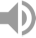DiscoverLearning Machines 101LM101-077: How to Choose the Best Model using BIC# LM101-077: How to Choose the Best Model using BIC

Update: 2019-05-02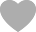1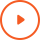Share

Description

In this 77th episode of www.learningmachines101.com , we explain the proper semantic interpretation of the Bayesian Information Criterion (BIC) and emphasize how this semantic interpretation is fundamentally different from AIC (Akaike Information Criterion) model selection methods. Briefly, BIC is used to estimate the probability of the training data given the probability model, while AIC is used to estimate out-of-sample prediction error. The probability of the training data given the model is called the “marginal likelihood”.  Using the marginal likelihood, one can calculate the probability of a model given the training data and then use this analysis to support selecting the most probable model, selecting a model that minimizes expected risk, and support Bayesian model averaging. The assumptions which are required for BIC to be a valid approximation for the probability of the training data given the probability model are also discussed.Comments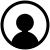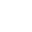00:00
00:00
1.0x

0.5x

0.8x

1.0x

1.25x

1.5x

2.0x

3.0x